# Real gas tables: isentropic work

kandelabr
Hello.

I am writing a Matlab program for calculating properties and processes of dry air using real gas tables. The only tables I could find were from Perry's Chemical Engineer's Handbook (7th edition, 1999).
Mostly I make use of cubic interpolation and in some critical cases numerical differentiation and integration of thermodynamic equations (for instance, calculating entropy from equation of state and cp tables).

First, I think I found a few mistakes in the tables and I guess there is even more of them.
This is a surface plot of cp (heat capacity at constant pressure); temperature 240-500 K and pressure 1 – 500 bar: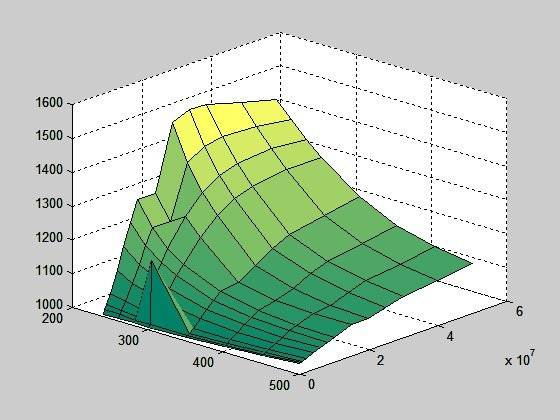If I filter the peak out and correct the two values where graph doesn't rise continuously (using interpolation), I get this: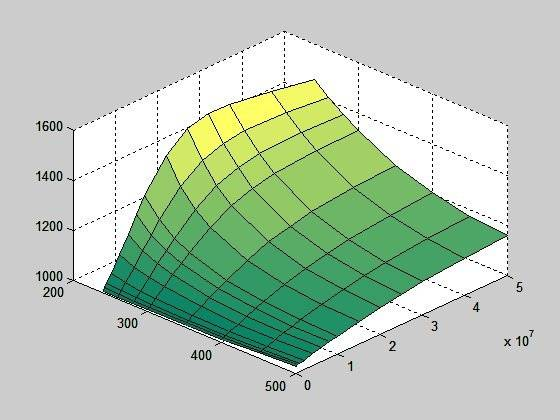But, if I calculate cp using equation cp = dh/dT (I also have an enthalpy table), the surface looks like this: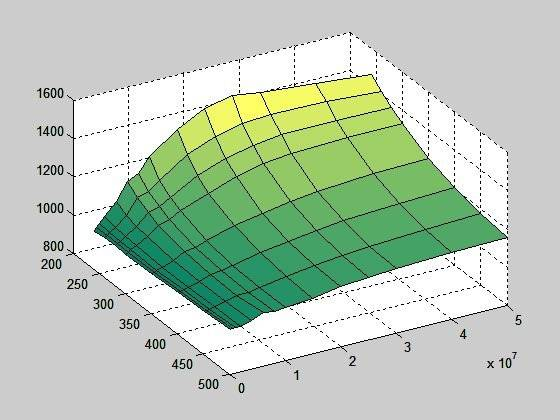Now there must be something wrong either with enthalpy table or cp table or with whatever table;
Second, I cannot calculate isentropic work of gas.
I am given the temperature and pressure at the beginning (T1, p1), and volume ratio (vr = v2/v1) (I am using volume ratio because I am developing a volumetric expander).

I tried 6 (six!) different approaches (I can post the code of all of them, If you want), but the results are definitely wrong since every method gives different results for the same start parameters.

The following graph shows work for T1 = 400K, vr = 2 and p1 goes from 15 bar to 500 bar.
The red method uses only equation of state (ideal gas equation and interpolation of factor of compressibility from tables) and a heap of numerical equation solving.
The blue is the most brutal way, which means using a while loop to add up temperatures bit by bit until the desired volume is reached.
Work of ideal gas would be drawn as a horizontal line at around 7x104 J/kg. Keep in mind that this is not one expansion but a series of expansions, each starting at a different pressure p1, which is on horizontal axis.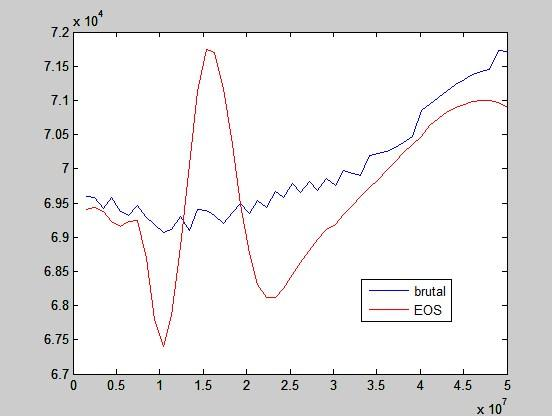This seems like a total nonsense. If it is of any help, this is the final temperature after expansions: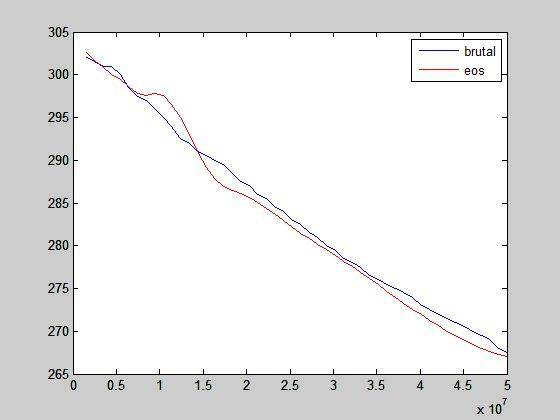So, my options are few:
- acquire better data from somewhere
- invent yet another method of calculating isentropic work (seems not a very good idea).

So, do you know where could I get thermodynamic properties of dry air? I only need volumes and specific heats (other tables would be welcome, though). Accuracy is critical!

Thank you,
kandelabr

## Answers and Replies

kandelabr
I hardly believe there is no one who has ever worked with real gases. How do you build a high pressure compressor? A diving cylinder? An air gun?
Using ideal gas and miss the numbers by 40%?

It's not only these forums. I can't find any data on the net (except that book), there is no book in our library (which is not too small), no real gas equations-of-state coefficients (Redlich-Kwong, Benedict-Webb-Rubin, virial equations), nothing, nothing. I feel like I was doing some basic research.

Homework Helper
Gold Member
I hardly believe there is no one who has ever worked with real gases. How do you build a high pressure compressor? A diving cylinder? An air gun?
Using ideal gas and miss the numbers by 40%?

It's not only these forums. I can't find any data on the net (except that book), there is no book in our library (which is not too small), no real gas equations-of-state coefficients (Redlich-Kwong, Benedict-Webb-Rubin, virial equations), nothing, nothing. I feel like I was doing some basic research.
Hi kandelabr,
The company I work for has their own thermophysical fluids database and I'm sure many other companies do also. But you can purchase a database from NIST called REFPROP for $200 or$300.
http://www.nist.gov/srd/nist23.cfm

I've used it and it's very similar to the one we have. You can link it to EXCEL and I believe to Matlab too, but I haven't used it with Matlab.

kandelabr